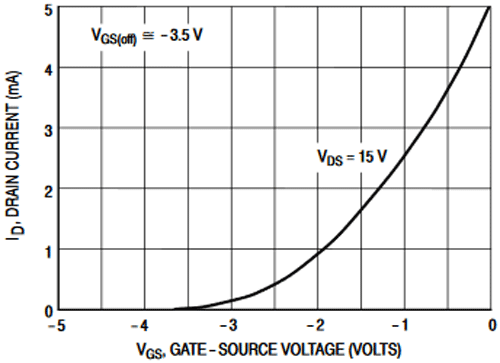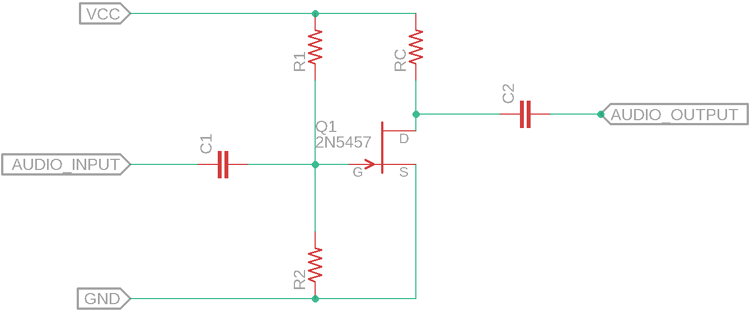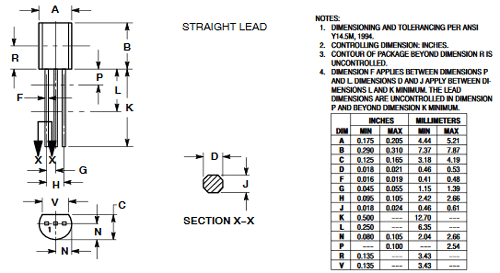# 2N5457 General Purpose N-Channel JFET

[Click the image to enlarge it]

2N5457 is an N-Channel Depletion layer Junction Field Effect Transistor. This JFET is an N- Channel - Depletion layer JFET that is widely used in audio equipment for amplification, tone modulation, and other purposes. It has a Drain-Source Voltage of 25 Vdc with a Drain-Gate voltage of 25 Vdc. The reverse Gate-Source Voltage is -25V.

### Pin Description of 2N5457 JFET

 Pin Number Pin Name Description 1 Drain Drain pin of the JFET 2 Source Source pin of the JFET 3 Gate Gate pin of the JFET

### Features and Specification of 2N5457 JFET

• Type: JFET - N - Channel - Depletion
• Segment: General Purpose
• Drain to Source Voltage (VDS)= 25V
• Drain to Gate Voltage (VDG)= 25V
• Gate to Source Voltage (VGS)= -25V
• Gate Current (IG)= 10 mA
• Gate-Source Cutoff Voltage - VGS (off) = -6.0 Vdc (VDS = 15 Vdc, ID = 10 nAdc)

Note: Complete technical details can be found in the 2N5457 Datasheet, linked at the bottom of this page.

### Alternative product of 2N5457 JFET

Other Replacements for 2N5457 JFET are 2N5458, J107, J309, BF246B, etc.

### 2N5457 JFET- Overview

The 2N5457 is an N-Channel JFET. It is suitable for general-purpose amplification and switching related applications. The below graph is the common source transfer graph, useful to know the common source transfer characteristic of the JFET. This JFET is also useful for switching related applications.### How to Select a JFET

1. Choose the JFET type that is required for the application. 2N5457 is an N - Channel JFET thus it is suitable to use on the low side of the power path. If the application requires high side switching, then use P-Channel.

2. Calculate the maximum Drain-Source voltage and the Gate-Source voltage will be for the targeted application. Choose a JFET that has the Drain-Source and Gate-Source breakdown voltage more than what is actually required. 2N5457 supports 25V Drain-Source breakdown voltage and -25V Gate-Source Breakdown voltage.

3. Calculate maximum Drain Current. Choose a JFET that could tolerate more than the Drain current that is actually required for the application. 2N5457 works up to 0.005A of continuous Drain current (ID).

### 2N5457 - Interfacing Diagram

The below image is showing the standard application of the N-Channel JFET 2N5457 as an amplifier.The C1 is the coupling capacitor and the C2 is the DC blocking capacitor where the output will be harvested. The RC is the collector Resistor and changing this value will change the frequency response of the circuit as well as it will control the DC gain by controlling the collector current. R1 and R2 are used as a voltage divider for biasing the JFET.

A typical value for 12V operation could be, R1 = 100k, R2 = 10k, RC = 10k, and C1, C2 can be 1uF ceramic capacitors.

### Applications of 2N5457 JFET

• Amplifier system
• Small switching Application
• Audio modulation
• Tone control
• Noise Generator

### 2D Model of 2N5457

The dimensions of 2N5457 are given below to help you with selecting the right PCB footprint for 2N5457.Component Datasheet